# 《最短路径·O(n^3)Floyd篇》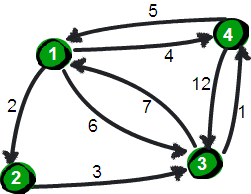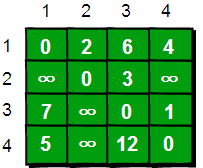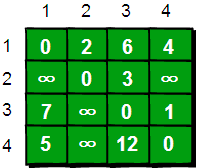for (i = 1; i <= n; i++)
{
for (j = 1; j <= n; j++)
{
if (a[i][j] > a[i] + a[j])
{
a[i][j] = a[i] + a[j];
}
}
}


for i:=1 to n do
begin
for j:=1 to n do
begin
if a[i,j]>a[i,1]+a[1,j] then
begin
a[i,j]:=a[i,1]+a[1,j];
end;
end;
end;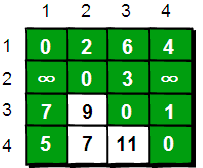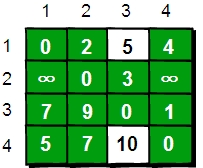同理，继续在只允许经过1、2和3号顶点进行中转的情况下，求任意两点之间的最短路程。任意两点之间的最短路程更新为：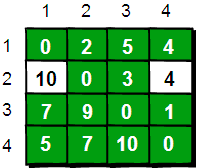WOW！出来了，这么说找中间点还得需要一个循环。但是，顺序该怎么打呢？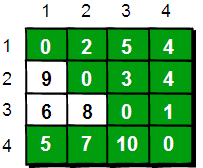for(k=1;k<=n;k++)
for(i=1;i<=n;i++)
for(j=1;j<=n;j++)
if(a[i][j]>a[i][k]+a[k][j])
a[i][j]=a[i][k]+a[k][j];


WOW！超短哟！这段代码的基本思想就是：最开始只允许经过1号顶点进行中转，接下来只允许经过1和2号顶点进行中转……允许经过1~n号所有顶点进行中转，求任意两点之间的最短路程。用一句话概括就是：从i号顶点到j号顶点只经过前k号点的最短路程。

k: 中间的过渡点
i: 前面的点
j: 后面的点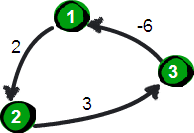# 《最短路径·O(n^3)Floyd篇》终！

©️2019 CSDN 皮肤主题: 数字20 设计师: CSDN官方博客